# Cleaning up spam

Hi everybody, in order to deal with the spam problem I have deleted all users regarded by WordPress as spam users. These are defined as users who have never posted. Unfortunately this seems to have caught some legitimate users as well. Their content is still on the blog, with the author marked as anonymous.

# Changes in the progressions

By popular demand Cathy Kessel has produced a document outlining the major changes in the recent version of the progressions.

# Modeling progression

Here is the almost final draft of the modeling progression.

# Almost final versions of progressions up through Ratios and Proportional Relationships

Cathy Kessel has been working hard to incorporate the feedback from this blog and from other places into final versions of the progressions. Here is the close to final version of the progressions up through Ratios and Proportional Relationships. Major edits include an expanded preface, a comprehensive introduction, and some significant additions to the fractions progression.

# Almost final version of fractions progression

Cathy Kessel is working on editing the progressions documents into final versions, incorporating comments from this blog and elsewhere and filling in gaps.  Here is the fractions progression. We will be rolling out the others over the next few months. Comments welcome!

# Meanwhile, over at the IM blog . . .

With the high school writing work in full swing, I’ve been remiss in posting here lately. I do have some ideas saved up! Meanwhile, Kate Nowak and I have a piece about instructional routines over at the IM blog.

# The Illustrative Mathematics blog

I’ve been meaning to let you know about the Illustrative Mathematics blog, which launched a few weeks ago. It has blog posts by members of the IM community about our grades 6–8 curriculum and about teaching practice, including a whole series on the 5 practices framework of Smith and Stein. Also, we will be cross posting any IM related posts I write here over there as well. I hope you find our new blog useful!

# Why is the graph of a linear function a straight line?

In my last post I wrote about the following standard, and mentioned that I could write a whole blog post about the first comma.

8.F.A.3. Interpret the equation $y = mx + b$ as defining a linear function, whose graph is a straight line; give examples of functions that are not linear. For example, the function $A = s^2$ giving the area of a square as a function of its side length is not linear because its graph contains the points $(1,1)$, $(2,4)$ and $(3,9)$, which are not on a straight line.

The comma indicates that the clause “whose graph is a straight line” is nonessential for identifying the noun phrase “linear function.” It turns the clause into an extra piece of information: “and by the way, did you know that the graph of a linear function is a straight line?” This fact is often presented as obvious; after all, if you draw the graph or produce it using a graphing utility, it certainly looks like a straight line.

When I’ve asked prospective teachers why this is so, I’ve gotten answers that look something like this:

We know that a linear function has a constant rate of change, $m$. If you go across by 1 on the graph you always go up by $m$, like this: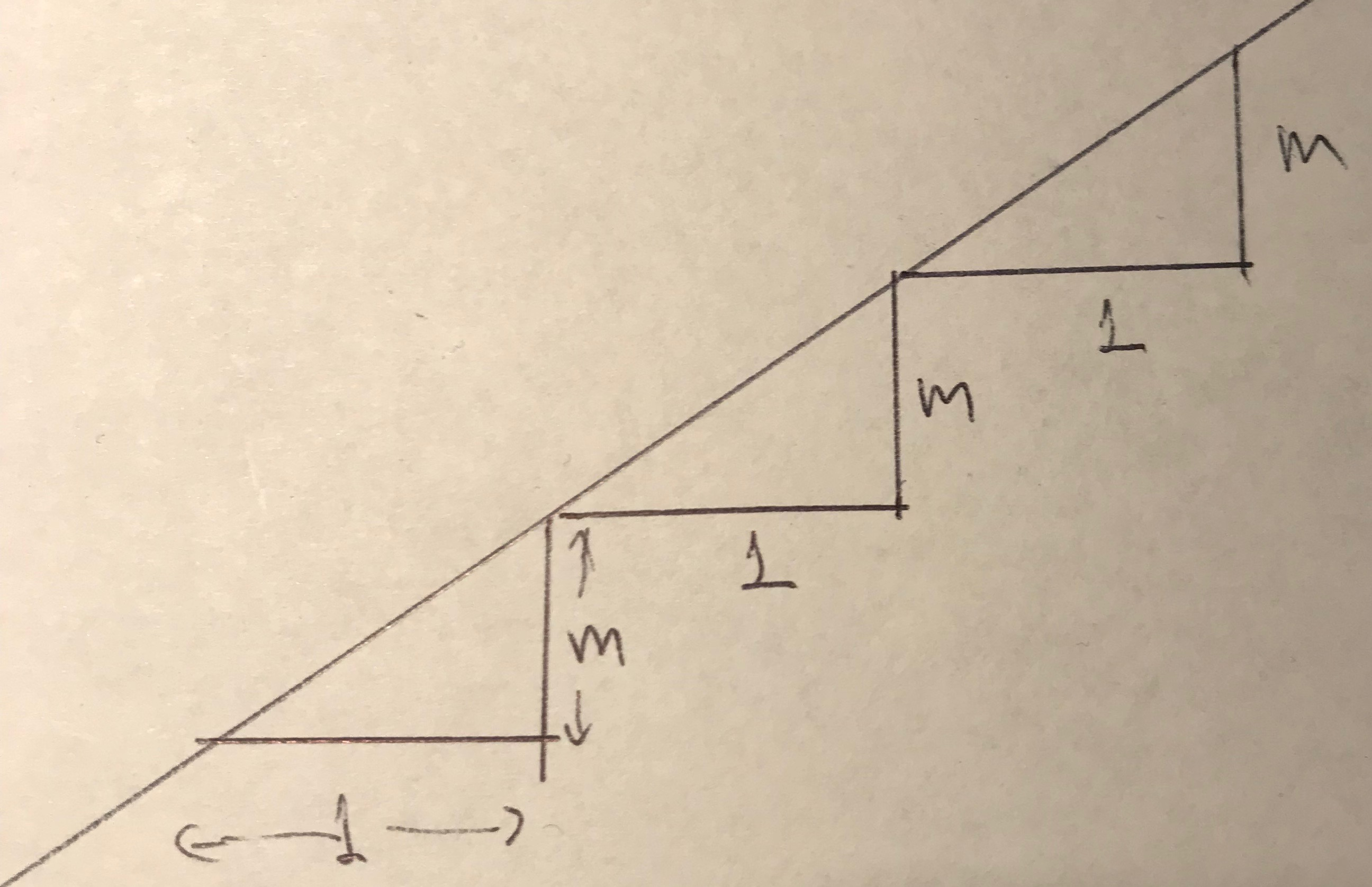So the graph is like a staircase. It always goes up in steps of the same size, so it’s a straight line.

This is fine as far as it goes. It identifies the defining property of a linear function—that it has a constant rate of change—and relates that property to a geometric feature of the graph. But it’s a “Here, Look!” proof. In the end it is showing that something is true rather than showing why it is true. Which is to say that it’s not a proof.

Still, the move to a geometric property of linear functions is a move in the right direction, because it focuses our minds on the essential concept. We all know that any two points lie on a line, but three points might not. What is it about three points on the graph of a linear function that implies they must lie on a straight line?Line from $A$ to $B$ to $C$ is dotted because we don’t know it’s a line yet

Because a linear function has a constant rate of change, the slope between any two of the three points $A$, $B$, and $C$ is the same. So $|BP|/|AP| = |CQ|/|AQ|$, which means there is a scale factor $k =|AQ|/|AP| = |CQ|/|BP|$ so that a dilation with center $A$ and scale factor $k$ takes $P$ to $Q$, and take the vertical line segment $BP$ to a vertical line segment based at $Q$ with the same length as $CQ$. Which means it must take $B$ to $C$.

But (drumroll) this means that there is a dilation with center $A$ that takes $B$ to $C$. Dilations always take points on a ray from the center to other points on the same ray. So $A$, $B$, and $C$ lie on the same line.

I don’t really expect students to get all of this, at least not right away. I’d be happy if they understood that there is a geometric fact at play here; that seeing is not always believing.

# How do you tell if a function is linear?

Over at the IM Virtual Math Coach we got a question about the following grade 8 standard:

8.F.A.3. Interpret the equation $y = mx + b$ as defining a linear function, whose graph is a straight line; give examples of functions that are not linear. For example, the function $A = s^2$ giving the area of a square as a function of its side length is not linear because its graph contains the points $(1,1)$, $(2,4)$ and $(3,9)$, which are not on a straight line.

I could write a whole blog post about that comma in the first sentence, but for now I want to focus on the question of what exactly are the student expectations entailed by this standard. Certainly students should be able to recognize that $y = mx + b$ defines a linear function; and they should be able to show a function is not linear by finding points on the graph with different slopes between them. (By definition, a linear function is one with a constant rate of change, that is, a function where the slope between any two points on its graph is always the same.) However, the following PARCC released item suggests the possible expectation that students be able to tell if a function is linear or not purely from looking at its defining equation.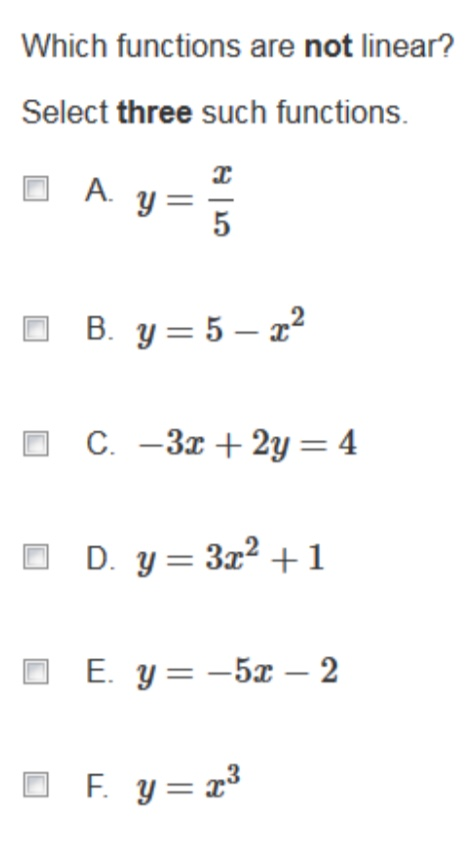My guess is that the writer of this item  was thinking that students would detect non-linear functions by noticing the functions with squared and cubed terms (they could also use the point method but that doesn’t seem likely). That’s not a good path to lead them down. Sure, $y = 5-x^2$ is not linear. What about $y = 5 – x^2 + (x+1)^2$? By the look of it it is even more not linear! But of course it is in fact linear, because the expression on the right is equivalent to $2x + 6$. The grade 8 standards don’t expect students to be making such simplifications as expanding squares of binomials.

This is another example of the confusion between expressions and functions. The expression $(x+1)^2 – x^2$ has non-linear terms in it, but the function it defines is linear because it is equivalent to $2x + 1$. Equivalent expressions define the same function.

There’s another confusion revealed in this item, the confusion between equations and functions. Look at option C. Is it intended to be a distractor? Will a student who chooses it be marked wrong? Such a student would have a case for protest on the grounds that $-3x + 2y = 4$ is not a linear function because it is not a function, it is an equation. Certainly if you choose to think of $x$ as the input and solve for $y$ to get the output you can think of it as a function, which would indeed be linear. You could also go the other way around and choose $y$ as the input and get a different linear function. It is conventional when $x$s and $y$s are floating around to think of $x$ as the input and $y$ as the output, but you can flout convention without being mathematically incorrect.

The moral of this story is, I suppose, that it is easier to tell when a function is not linear than to tell when it is linear. Testing for non-linearity involves just picking a few points on the graph; testing for linearity involves picking every possible pair of points on the graph and verifying that the slope between them is always the same. It’s instructive to do this with $y = mx +b$: if $(x_1,y_1)$ and $(x_2,y_2)$ are two different ordered pairs satisfying this equation, then $$\frac{y_2-y_1}{x_2-x_1} = \frac{{mx_2 + b} – (mx_1 +b)}{x_2-x_1} = \frac{m(x_2-x_1)}{x_2-x_1} = m.$$

OK, next week I’ll write a blog post about the comma.

# The image of function in school mathematics

Well, oops, took a little break from blogging there. But I’m back now.

In the course of working on an article with the same title as this blog post for a publication about Felix Klein, I did a Google Image search on the word “function,” with the following results.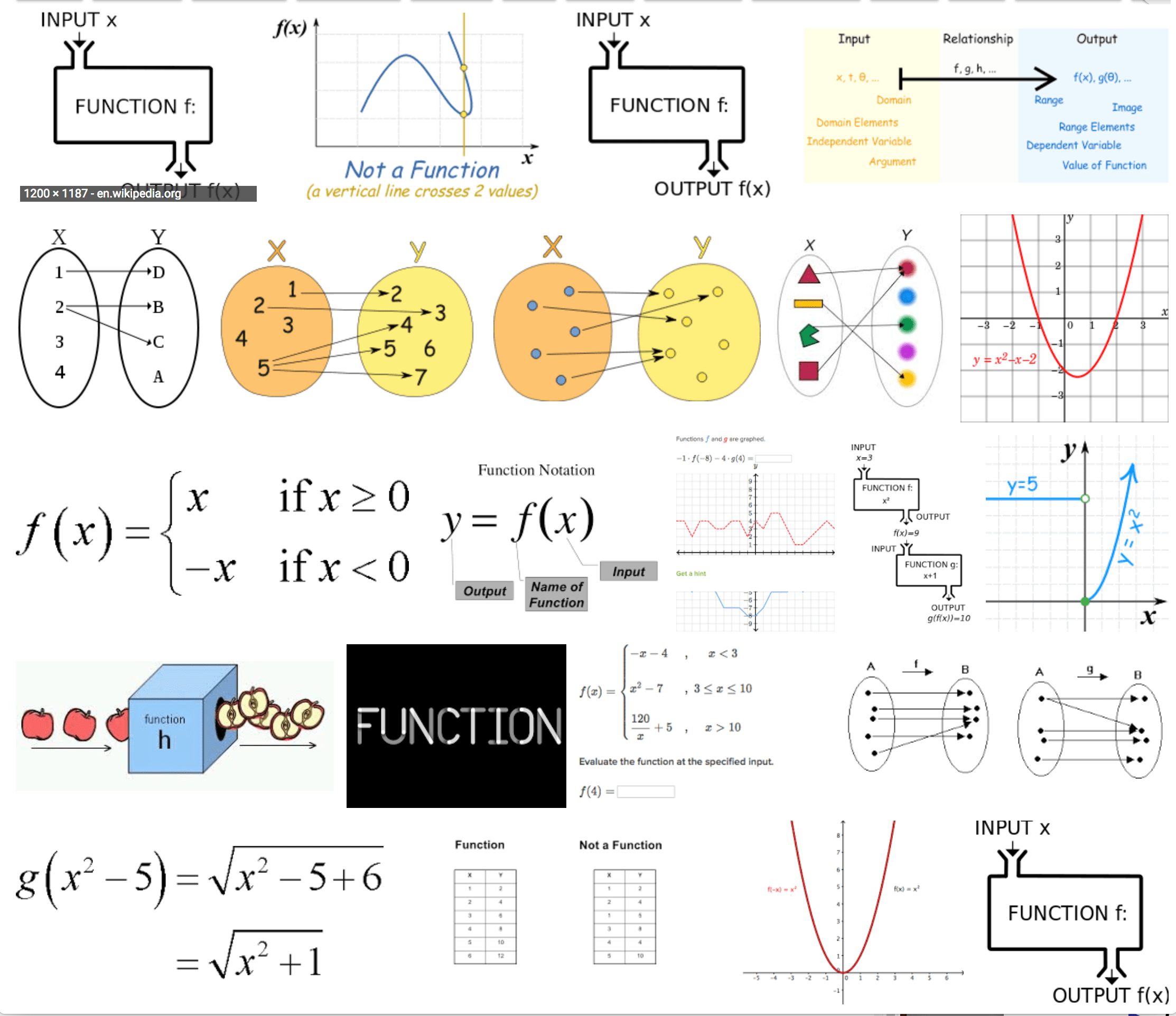I find this fascinating for a number of reason. First, notice the proliferation of representations: graphs, tables, formulas, input-output machines, and arrow diagrams (those blobs in the second row). A corresponding search in a French, German, or Japanese gives a very different result. Here is the Japanese one (where I searched google.co.jp for “関数”).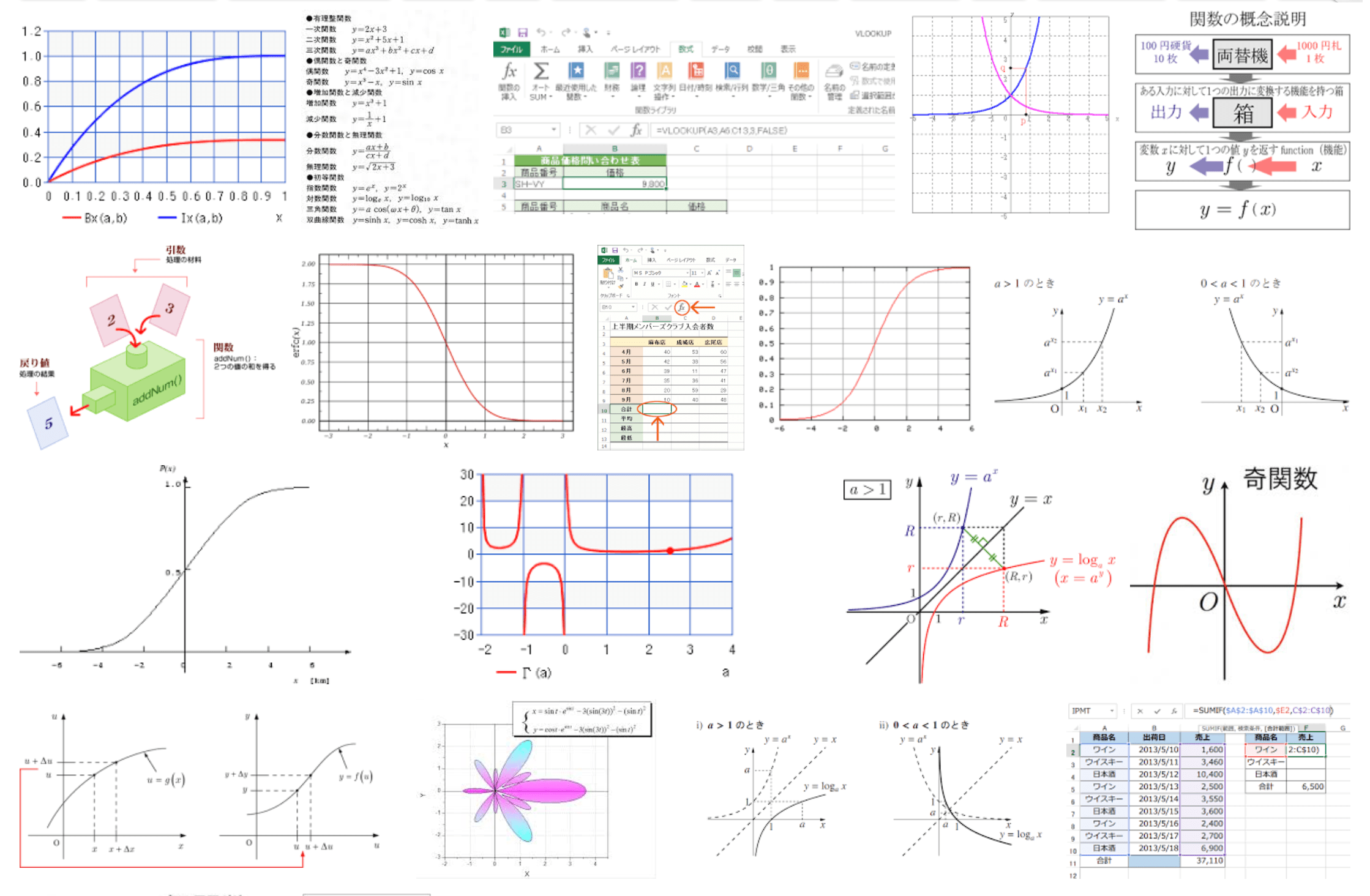This has a much greater focus on graphs, and no arrow diagrams. The French and German ones are even more focused on graphs; a search in Spanish, however, gives results similar to English.

What does all this mean? Well, I’ll be discussing this more in the article I’m writing, but for now I have just a couple of observations.

First, the English language search reveals a preoccupation with examples of relations that are not functions, with examples of graphs, arrow diagrams, and tables. I have always found this preoccupation perverse: the examples are artificial, and there is very little to be gained from them except an ability to answer questions about them on tests. This preoccupation is not evident in the searches in other languages.

Second, take a look at these two representations, from the English and Japanese language searches: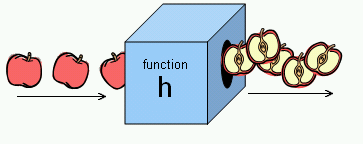.

Together they represent a case study in designing representations. Can you see what’s wrong with the one on the top? A function is supposed to have only one output for every input. Does an apple slicing machine have that property?* The representation on the bottom, on the other hand, clearly represents how to think of addition as a function with two inputs.

I’m interested to hear readers’ thoughts on the representations that come up in these and other searches. Maybe someone who can navigate Baidu can tell us what the Chinese results are.

*To be fair, the authors of the web page with the apple slicing machine are clearly aware of the problem. But their contortions to get around it only emphasize the fundamental flaws in the representation.